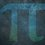# UKMT Special (Problem $19$)

Solve the pair of simultaneous equations:

$x^2 + 3y = 10$

$3 + y = \frac{10}{x}$

[UKMT Hamilton Olympiad $2017$, H$6$]Note by Yajat Shamji
8 months, 2 weeks ago

This discussion board is a place to discuss our Daily Challenges and the math and science related to those challenges. Explanations are more than just a solution — they should explain the steps and thinking strategies that you used to obtain the solution. Comments should further the discussion of math and science.

When posting on Brilliant:

• Use the emojis to react to an explanation, whether you're congratulating a job well done , or just really confused .
• Ask specific questions about the challenge or the steps in somebody's explanation. Well-posed questions can add a lot to the discussion, but posting "I don't understand!" doesn't help anyone.
• Try to contribute something new to the discussion, whether it is an extension, generalization or other idea related to the challenge.

MarkdownAppears as
*italics* or _italics_ italics
**bold** or __bold__ bold
- bulleted- list
• bulleted
• list
1. numbered2. list
1. numbered
2. list
Note: you must add a full line of space before and after lists for them to show up correctly
paragraph 1paragraph 2

paragraph 1

paragraph 2

[example link](https://brilliant.org)example link
> This is a quote
This is a quote
    # I indented these lines
# 4 spaces, and now they show
# up as a code block.

print "hello world"
# I indented these lines
# 4 spaces, and now they show
# up as a code block.

print "hello world"
MathAppears as
Remember to wrap math in $$ ... $$ or $ ... $ to ensure proper formatting.
2 \times 3 $2 \times 3$
2^{34} $2^{34}$
a_{i-1} $a_{i-1}$
\frac{2}{3} $\frac{2}{3}$
\sqrt{2} $\sqrt{2}$
\sum_{i=1}^3 $\sum_{i=1}^3$
\sin \theta $\sin \theta$
\boxed{123} $\boxed{123}$

Sort by:

You have until next Tuesday, $3:00$pm!

- 8 months, 2 weeks ago

Step 1) Substitute y

   Equation 2    =>    y = 10/x - 3
Equation 1     =>    x^2 + 3(10/x -3) = 10
=>    x^2 + 30/x - 9 = 10
=>    x^2 + 30/x - 19 = 0
=>    x^3 - 19x + 30 = 0


Step 2) Factor the cubic Let P(x) = x^3 - 19x + 30

   According to rational root theorem, the only rational root possible is
(x +- 30), (x +- 15), (x +- 10), (x +- 5), (x +- 3), (x +- 2), (x +- 1)

According to polynomial factor theorem, if P(a) = 0, then (x - a) is a root.
We try all the possible rational factor.
P(-5) = P(2) = P(3) = 0

We can conclude that P(x) = (x + 5)(x - 2)(x - 3)


Step 3) Finding x and y x^3 - 19x + 30 = 0 (x + 5)(x - 2)(x - 3) = 0 x = -5 or x = 2 or x = 3

 Now, find y by substituting x to equation 2.
x = -5   =>    y = -5
x = 2    =>    y = 2
x = 3    =>    y = 1/3


The solutions are (x,y) = {(-5, -5), (2, 2), (3, 1/3)}

- 8 months, 2 weeks ago